# LIC AAO/SBI PO Prelims Quantitative Aptitude Questions 2019 (Day-03)

Dear Aspirants, Our IBPS Guide team is providing new series of Quantitative Aptitude Questions for LIC AAO/SBI PO 2019 so the aspirants can practice it on a daily basis. These questions are framed by our skilled experts after understanding your needs thoroughly. Aspirants can practice these new series questions daily to familiarize with the exact exam pattern and make your preparation effective.

[WpProQuiz 5493]

### Click Here for SBI PO Pre 2019 High-Quality Mocks Exactly on SBI Standard

Directions (1 – 5): The questions below are based on the given Series-I. The series-I satisfy a certain pattern, follow the same pattern in Series-II and find the wrong number in the series –II.

1)

I) 112, 233, 1961, 2130, 4874, 5099

II) 256, 377, 2103, 2274, 5018, 5243

a) 5018

b) 377

c) 2103

d) 256

e) 2274

2)

I) 52, 26, 39, 97.5, 341.25

II) 128, 64, 96, 238, 840

a) 238

b) 64

c) 96

d) 840

e) 128

3)

I) 384, 556, 729, 929, 1254, 1922

II) 192, 364, 537, 737, 1062, 1728

a) 364

b) 1728

c) 192

d) 737

e) 1062

4)

I) 12, 14, 26, 56, 112, 202, 334

II) 23, 25, 37, 67, 125, 213, 345

a) 345

b) 125

c) 25

d) 67

e) 213

5)

I) 236, 236, 243, 217, 280, 156

II) 384, 384, 390, 365, 428, 304

a) 384

b) 365

c) 390

d) 304

e) 428

Directions (6 – 10): Study the following information carefully and answer the questions given below.

The following table shows the total number of people working in various departments of different organizations in particular city.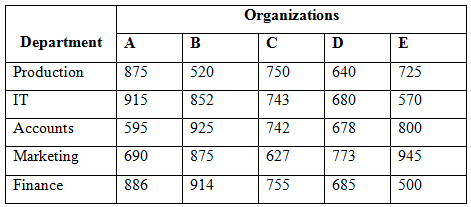6) Total number of employees working in Accounts department is approximately what percentage of total number of employees working in marketing department of all the given organizations?

a) 84 %

b) 96 %

c) 75 %

d) 108 %

e) 117 %

7) Find the ratio between the total number of employees working in organization C to that of D of all the given department together?

a) 1156: 1027

b) 2458: 2175

c) 987: 756

d) 3617: 3456

e) None of these

8) Total number of employees working in IT and Finance of Organization A, C and E together is approximately what percentage more/less than the total number of employees working in Production and Marketing of Organization B, D and E together?

a) 2 % more

b) 15 % less

c) 2 % less

d) 15 % more

e) 23 % less

9) Find the difference between the total number of employees working in Organization B to that of E of all the given department together?

a) 546

b) 678

c) 424

d) 712

e) None of these

10) Which of the following Organization has highest number of employees?

a) Organization A

b) Organization B

c) Organization C

d) Organization D

e) Organization E

Series I pattern: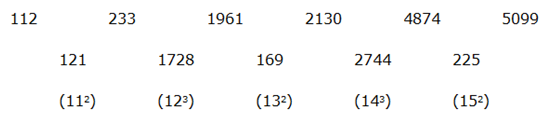Series II pattern: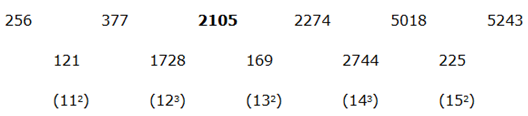The wrong term is, 2103

Series I pattern:

52* 0.5 = 26

26* 1.5 = 39

39* 2.5 = 97.5

97.5* 3.5 = 341.25

Series II pattern:

128*0.5 = 64

64* 1.5 = 96

96* 2.5 = 240

240*3.5 = 840

The wrong term is, 238

Series I pattern: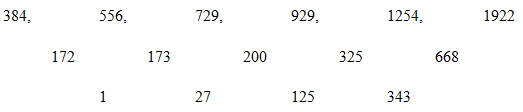The difference of difference is, 13, 33, 53, 73,…

Series II pattern: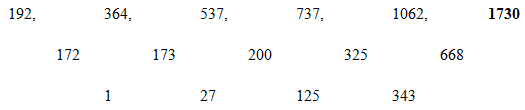The wrong term is, 1728

Series I pattern:

12 + (1*2) = 14

14 + (3*4) = 26

26 + (5*6) = 56

56 + (7*8) = 112

112 + (9*10) = 202

202 + (11*12) = 334

Series II pattern:

23 + (1*2) = 25

25 + (3*4) = 37

37 + (5*6) = 67

67 + (7*8) = 123

123 + (9*10) = 213

213 + (11*12) = 345

The wrong term is, 125

Series I pattern:

236 – (13 – 1) = 236

236 + (23 – 1) = 243

243 – (33 – 1) = 217

217 + (43 – 1) = 280

280 – (53 – 1) = 156

Series II pattern:

384 – (13 – 1) = 384

384 + (23 – 1) = 391

391 – (33 – 1) = 365

365 + (43 – 1) = 428

428 – (53 – 1) = 304

The wrong term is, 390

Total number of employees working in Account department

= > 595 + 925 + 742 + 678 + 800

= > 3740

Total number of employees working in marketing department

= > 690 + 875 + 627 + 773 + 945

= > 3910

Required %= (3740/3910)*100 = 95.65 % = 96 %

The total number of employees working in organization C

= > 750 + 743 + 742 +627 + 755

= > 3617

The total number of employees working in organization D

= > 640 + 680 + 678 + 773 + 685

= > 3456

Required ratio = 3617: 3456

Total number of employees working in IT and Finance of Organization A, C and E together

= > 915 + 743 + 570 + 886 + 755 + 500

= > 4369

Total number of employees working in Production and Marketing of Organization B, D and E together

= > 520 + 640 + 725 + 875 + 773 + 945

= > 4478

Required % = [(4478 – 4369)/4478]*100 = (109/4478)*100

= > 2.434 % = 2 % less

The total number of employees working in Organization B

= > 520 + 852 + 925 + 875 + 914

= > 4086

The total number of employees working in Organization E

= > 725 + 570 + 800 + 945 + 500

= > 3540

Required difference = 4086 – 3540 = 546

Total number of employees in Organization A

= > 875 + 915 + 595 + 690 + 886

= > 3961

Total number of employees in Organization B

= > 520 + 852 + 925 + 875 + 914

= > 4086

Total number of employees in Organization C

= > 750 + 743 + 742 + 627 + 755

= > 3617

Total number of employees in Organization D

= > 640 + 680 + 678 + 773 + 685

= > 3456

Total number of employees in Organization E

= > 725 + 570 + 800 + 945 + 500

= > 3540

Organization B has highest number of employees.